1289766752

• 2

获得赞
• 2

发布的文章
• 0

答辩的项目

## pointnet与pointnet++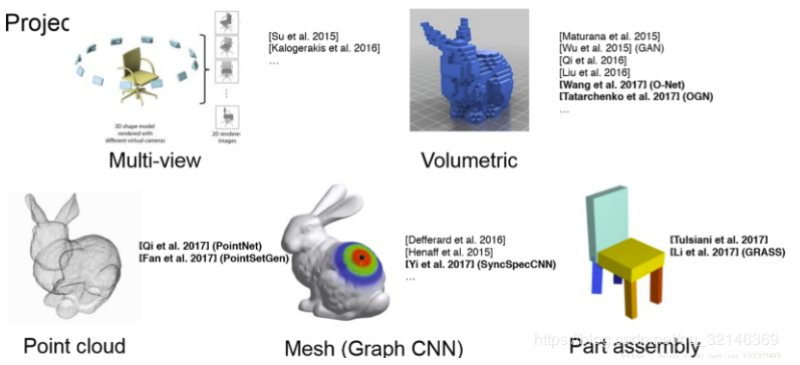二、点云的问题  无序性：点云本质上是一长串点（nx3矩阵，其中n是点数）。在几何上，点的顺序不影响它在空间中对整体形状的表示，例如，相同的点云可以由两个完全不同的矩阵表示。 希望得到的效果如下图右边：N代表点云个数，D代表每个点的特征维度。不论点云顺序怎样，希望得到相同的特征提取结果。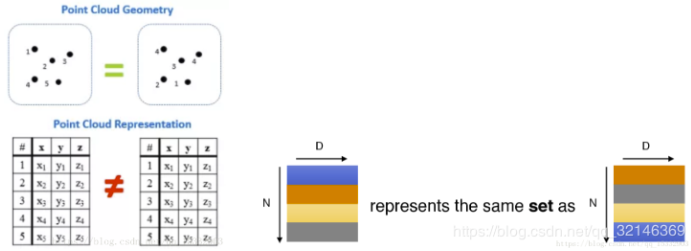网络的一般结构是：提特征-特征映射-特征图压缩（降维）-全连接。  下图中x代表点云中某个点，h代表特征提取层，g叫做对称方法，r代表更高维特征提取，最后接一个softmax分类。g可以是maxpooling或sumpooling，也就是说，最后的D维特征对每一维都选取N个点中对应的最大特征值或特征值总和，这样就可以通过g来解决无序性问题。pointnet采用了max-pooling策略。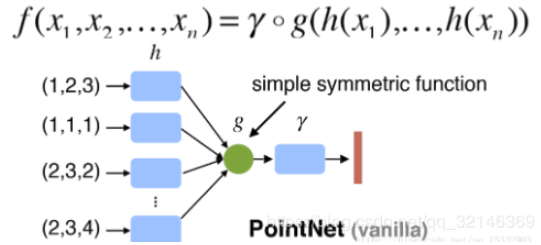2.旋转性：  点云的旋转不变性指的是，给予一个点云一个旋转，所有的x,y,z x,y,zx,y,z坐标都变了，但是代表的还是同一个物体。  ​    ​  因此对于普通的PointNet(vanilla)，如果先后输入同一个但是经过不同旋转角度的物体，它可能不能很好地将其识别出来。在论文中的方法是新引入了一个T-Net网络去学习点云的旋转，将物体校准，剩下来的PointNet(vanilla)只需要对校准后的物体进行分类或者分割即可。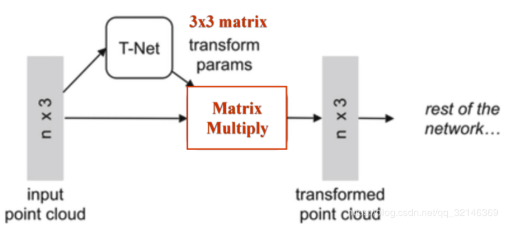由图可以看出，由于点云的旋转非常的简单，只需要对一个N×D的点云矩阵乘以一个D×D 的旋转矩阵即可，因此对输入点云学习一个3×3 的矩阵，即可将其矫正；同样的将点云映射到K维的冗余空间后，再对K维的点云特征做一次校对，只不过这次校对需要引入一个正则化惩罚项，希望其尽可能接近于一个正交矩阵。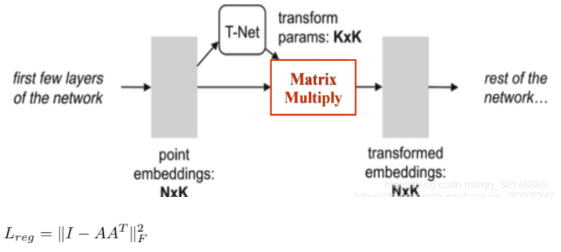三、pointnet网络结构详解   网络的两个亮点：  空间变换网络解决旋转问题：三维的STN可以通过学习点云本身的位姿信息学习到一个最有利于网络进行分类或分割的DxD旋转矩阵（D代表特征维度，pointnet中D采用3和64）。至于其中的原理，我的理解是，通过控制最后的loss来对变换矩阵进行调整，pointnet并不关心最后真正做了什么变换，只要有利于最后的结果都可以。pointnet采用了两次STN，第一次input transform是对空间中点云进行调整，直观上理解是旋转出一个更有利于分类或分割的角度，比如把物体转到正面；第二次feature transform是对提取出的64维特征进行对齐，即在特征层面对点云进行变换。 maxpooling解决无序性问题：网络对每个点进行了一定程度的特征提取之后，maxpooling可以对点云的整体提取出global feature。 网络结构：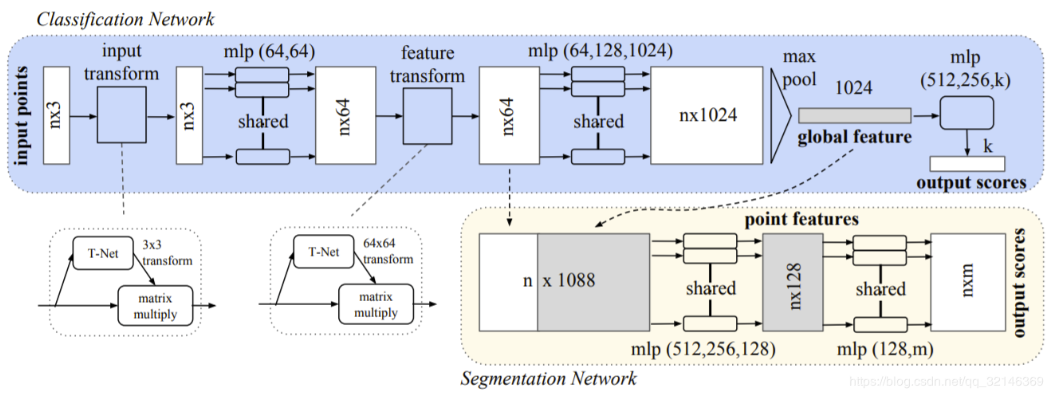其中，mlp是通过共享权重的卷积实现的，第一层卷积核大小是1x3（因为每个点的维度是xyz），之后的每一层卷积核大小都是1x1。即特征提取层只是把每个点连接起来而已。经过两个空间变换网络和两个mlp之后，对每一个点提取1024维特征，经过maxpool变成1x1024的全局特征。再经过一个mlp（代码中运用全连接）得到k个score。分类网络最后接的loss是softmax。       具体来说，对于每一个N×3 的点云输入，网络先通过一个T-Net将其在空间上对齐（旋转到正面），再通过MLP将其映射到64维的空间上，再进行对齐，最后映射到1024维的空间上。这时对于每一个点，都有一个1024维的向量表征，而这样的向量表征对于一个3维的点云明显是冗余的，因此这个时候引入最大池化操作，将1024维所有通道上都只保留最大的那一个，这样得到的1×1024 的向量就是N个点云的全局特征。  如果做的是分类的问题，直接将这个全局特征再进过MLP去输出每一类的概率即可；但如果是分割问题，由于需要输出的是逐点的类别，因此其将全局特征拼接在了点云64维的逐点特征上，最后通过MLP，输出逐点的分类概率。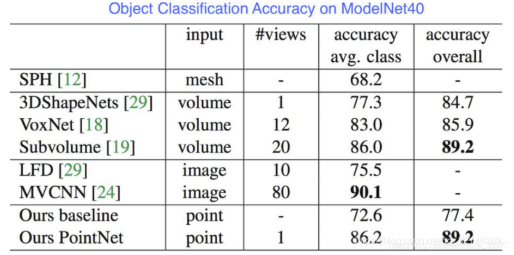不仅如此，论文对PointNet对点云的点的缺失鲁棒性做了实验，实验证明，当PointNet缺失了60%左右的点的时候，其网络的效果都不怎么减少：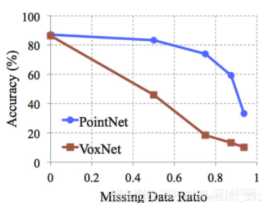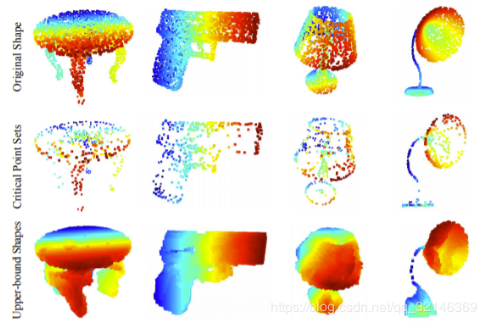```class T_Net(nn.Module):
def __init__(self):
super(T_Net, self).__init__()
# 这里需要注意的是上文提到的MLP均由卷积结构完成
# 比如说将3维映射到64维，其利用64个1x3的卷积核
self.conv1 = torch.nn.Conv1d(3, 64, 1)
self.conv2 = torch.nn.Conv1d(64, 128, 1)
self.conv3 = torch.nn.Conv1d(128, 1024, 1)
self.fc1 = nn.Linear(1024, 512)
self.fc2 = nn.Linear(512, 256)
self.fc3 = nn.Linear(256, 9)
self.relu = nn.ReLU()

self.bn1 = nn.BatchNorm1d(64)
self.bn2 = nn.BatchNorm1d(128)
self.bn3 = nn.BatchNorm1d(1024)
self.bn4 = nn.BatchNorm1d(512)
self.bn5 = nn.BatchNorm1d(256)

def forward(self, x):
batchsize = x.size()
x = F.relu(self.bn1(self.conv1(x)))
x = F.relu(self.bn2(self.conv2(x)))
x = F.relu(self.bn3(self.conv3(x)))
x = torch.max(x, 2, keepdim=True)
x = x.view(-1, 1024)

x = F.relu(self.bn4(self.fc1(x)))
x = F.relu(self.bn5(self.fc2(x)))
x = self.fc3(x)

iden = Variable(torch.from_numpy(np.array([1,0,0,0,1,0,0,0,1]).astype(np.float32))).view(1,9).repeat(batchsize,1)
if x.is_cuda:
iden = iden.cuda()
x = x + iden
x = x.view(-1, 3, 3) # 输出为Batch*3*3的张量
return x
```

PointNet的主体：

```class PointNetEncoder(nn.Module):
def __init__(self, global_feat = True):
super(PointNetEncoder, self).__init__()
self.stn = T_Net()
self.conv1 = torch.nn.Conv1d(3, 64, 1)
self.conv2 = torch.nn.Conv1d(64, 128, 1)
self.conv3 = torch.nn.Conv1d(128, 1024, 1)
self.bn1 = nn.BatchNorm1d(64)
self.bn2 = nn.BatchNorm1d(128)
self.bn3 = nn.BatchNorm1d(1024)
self.global_feat = global_feat
def forward(self, x):
'''生成全局特征'''
n_pts = x.size()
trans = self.stn(x)
x = x.transpose(2,1)
x = torch.bmm(x, trans) # batch matrix multiply 即乘以T-Net的结果
x = x.transpose(2,1)
x = self.conv1(x)

x = F.relu(self.bn1(x))
pointfeat = x
x_skip = self.conv2(x)

x = F.relu(self.bn2(x_skip))
x = self.bn3(self.conv3(x))
x = torch.max(x, 2, keepdim=True)
x = x.view(-1, 1024)
if self.global_feat:
return x, trans
else:
x = x.view(-1, 1024, 1).repeat(1, 1, n_pts)

class PointNetCls(nn.Module):
def __init__(self, k = 2):
super(PointNetCls, self).__init__()
self.k = k
self.feat = PointNetEncoder(global_feat=False)
self.conv1 = torch.nn.Conv1d(1088, 512, 1)
self.conv2 = torch.nn.Conv1d(512, 256, 1)
self.conv3 = torch.nn.Conv1d(256, 128, 1)
self.conv4 = torch.nn.Conv1d(128, self.k, 1)
self.bn1 = nn.BatchNorm1d(512)
self.bn2 = nn.BatchNorm1d(256)
self.bn3 = nn.BatchNorm1d(128)

def forward(self, x):
'''分类网络'''
batchsize = x.size()
n_pts = x.size()
x, trans = self.feat(x)
x = F.relu(self.bn1(self.conv1(x)))
x = F.relu(self.bn2(self.conv2(x)))
x = F.relu(self.bn3(self.conv3(x)))
x = self.conv4(x)
x = x.transpose(2,1).contiguous()
x = F.log_softmax(x.view(-1,self.k), dim=-1)
x = x.view(batchsize, n_pts, self.k)
return x

class PointNetPartSeg(nn.Module):
def __init__(self,num_class):
super(PointNetPartSeg, self).__init__()
self.k = num_class
self.feat = PointNetEncoder(global_feat=False)
self.conv1 = torch.nn.Conv1d(1088, 512, 1)
self.conv2 = torch.nn.Conv1d(512, 256, 1)
self.conv3 = torch.nn.Conv1d(256, 128, 1)
self.conv4 = torch.nn.Conv1d(128, self.k, 1)
self.bn1 = nn.BatchNorm1d(512)
self.bn1_1 = nn.BatchNorm1d(1024)
self.bn2 = nn.BatchNorm1d(256)
self.bn3 = nn.BatchNorm1d(128)

def forward(self, x):
'''分割网络'''
batchsize = x.size()
n_pts = x.size()
x, trans = self.feat(x)
x = F.relu(self.bn1(self.conv1(x)))
x = F.relu(self.bn2(self.conv2(x)))
x = F.relu(self.bn3(self.conv3(x)))
x = self.conv4(x)
x = x.transpose(2,1).contiguous()
x = F.log_softmax(x.view(-1,self.k), dim=-1)
x = x.view(batchsize, n_pts, self.k)
return x, trans
```

500字1289766752

• 2

获得赞
• 2

发布的文章
• 0

答辩的项目